# An eulerian-lagrangian scheme for the problem of the inverse design of hyperbolic transport equations

Authors: - 18 February 2020

The inverse design of hyperbolic transport equations can be addressed by using gradient-adjoint methodologies. Recently, Morales-Hernandez and Zuazua  investigated the convenience of using low order numerical schemes for the adjoint resolution in the gradient-adjoint method. They focused on hyperbolic transport scalar equations with an heterogeneous time-independent vector field.

Morales-Hernandez and Zuazua analysed the numerical resolution of the adjoint equation by means of two methods: a First Order Upwind $(FOU)$ scheme and a Second Order Upwind $(SOU)$ scheme. They concluded that the $FOU$ scheme is the best choice when dealing with smooth functions once the $SOU$ scheme introduces some spurious oscillations. In addition, the $FOU$ scheme requires shorter computational time than the $SOU$ scheme. The non-linear transport problems in which sharp interfaces or discontinuities may appear in the functions has remained to be studied.

The numerical scheme for the adjoint equation determines the direction of descent in the iteration procedure, and consequently the $CPU$ time consumed by the solver. As the $CPU$ time is a decisive factor, we propose an eulerian-lagrangian scheme that uses the Modified Method of Characteristics ($MMOC$)  for solving the transport and adjoint equations. The $MMOC$ method is based on the characteristic curves, and so very computationally competitive for linear hyperbolic transport equations. The $MMOC$ was initially developed performing the resolution in a forward sense. In this work, the eulerian-lagrangian scheme is used for the transport and adjoint resolution, employing it in both forward and backward senses.

We perform numerical tests in order to investigate the accuracy and efficiency of the eulerian-lagrangian scheme to solve the problem of inverse design of linear and non-linear hyperbolic transport scalar equations.

# The gradient-adjoint method

Consider the following hyperbolic transport scalar equation with a vector flux function ${\bf f} (u)$:

\begin{equation}\label{eq1} \frac{\partial u}{\partial t} + \nabla \cdot {\bf f} (u) = 0, ~ ~ u({\bf x},0) = u_0. \end{equation}

Given a target function $u^* \in \bf H$, $\bf H$ being a Hilbert space, the problem of inverse design consists of achieving $u_0 \in \bf H$ such that the solution of the former evolution equation satisfies $u(T) = u^*$. This problem is solved via the minimization of the functional

\begin{equation}\label{eq2} J(u_0) = \frac{1}{2} \int_{0}^{T} \int_{\Omega} (u(T) - u^*)^2 d\Omega dt \end{equation}

by means of the gradient descent method:

\begin{equation}\label{eq3} {u_0}^{k+1} = {u_0}^k - \epsilon {\nabla J}^k, \end{equation}

where $\epsilon$ is the step size, and the gradient $\nabla J = \sigma (0)$, in which $\sigma$ is the adjoint variable . The gradient $\sigma (0)$ is achieved by solving the adjoint equation:

The gradient-adjoint method is based on iterating a loop, where the transport equation (\ref{eq1}) is solved in a forward sense, while the adjoint equation (\ref{eq4}), which is of hyperbolic nature as well, is solved backwards in time.

# The $MMOC$ in forward sense

Eulerian-lagrangian approaches provide computationally efficient techniques for approximating the solution of hyperbolic transport equations. Douglas and Russel  introduced an eulerian-lagrangian method based on the characteristic curve - the $MMOC$ - to develop fast solvers for hyperbolic transport equations. Despite the $MMOC$ does not provide a local conservation of the identity associated with the function, it present good performance for linear hyperbolic transport equations.

We use the $MMOC$ method for solving the transport and adjoint equations in the context of the problem of inverse design of hyperbolic transport equations. In this problem, the adjoint equation is always a linear differencial equation, worthing the use of the eulerian-lagrangian scheme. The gradient-adjoint iteration requires the adjoint equation to be solved in backward sense. We employ an eulerian-lagrangian scheme that uses the $MMOC$, considering procedures in forward and backward senses.

Consider a scalar hyperbolic transport equation determining the initial value problem

\begin{equation}\label{eq5} \frac{\partial u}{\partial t} + \nabla \cdot {\bf f} (u) = 0, ~ ~ u({\bf x},0) = u_0 , \end{equation}

where ${\bf f} (u)$ is a vector flux function. Rewriting the Eq. (\ref{eq5}) in nondivergence form,

\begin{equation}\label{eq6} \frac{\partial u}{\partial t} + {\bf f}^\prime (u) \cdot \nabla u = 0 . \end{equation}

The solution of Eq. (\ref{eq6}) is essentially along the characteristic curves of the transport operator $\partial / \partial t + {\bf f}^\prime (u) \cdot \nabla$, so that it is appropriate to introduce differentiation in this characteristic direction. Let

\begin{equation}\label{eq7} \partial / \partial t + {\bf f}^\prime (u) \cdot \nabla = \psi \frac{\partial}{\partial \tau} , ~~\psi (u) = \sqrt {1 + {\left \lVert {\bf f}^\prime (u) \right \rVert}^2} ,
\end{equation}

in which the direction $\tau$ depends on $\bf x$.

Let us consider the discretization of Eq. (\ref{eq6}) in time. Denote the time step $\Delta t > 0$ and consider the approximation of the solution at times $t^n = n \Delta t$. In the standard $MMOC$, the characteristic derivative is approximated by

\begin{equation}\label{eq8} \psi \frac{\partial u}{\partial \tau} \approx \psi (u({\bf x}, t^{n-1})) \frac {u({\bf x}, t^{n}) - u(\overline {\bf x}^n, t^{n-1})}{\sqrt {\left \lVert {\bf x} - \overline {\bf x}^n \right \rVert^2 + (\Delta t)^2 }} = \frac {u({\bf x}, t^{n}) - u(\overline {\bf x}^n, t^{n-1})}{\Delta t} ,
\end{equation}

where

\begin{equation}\label{eq9} \overline {\bf x}^n = \overline {\bf x}^n ({\bf x}) = {\bf x} - {\bf f}^\prime (u({\bf x}, t^{n-1}) \Delta t . \end{equation}

Let $U^n ({\bf x})$ denote the approximations to $u({\bf x},t^n)$. For a predecessor position

\begin{equation}\label{eq10} \overline {\bf X}^n ({\bf x}) = {\bf x} - {\bf f}^\prime (U^{n-1} ({\bf x})) \Delta t, \end{equation}

the transported function is defined as

\begin{equation}\label{eq11} \overline {U}^n ({\bf x}) = u (\overline {\bf X}^n ({\bf x}), t^{n-1}) . \end{equation}

As the continuous in space approximation for Eq. (\ref{eq6}) is

\begin{equation}\label{eq12} \frac{U^n ({\bf x}) - \overline U^{n} ({\bf x})}{\Delta t} = 0 , \end{equation}

the $MMOC$ scheme is given by

# Preliminary results

The performance of the $MMOC$ scheme is checked in numerical tests, where two hyperbolic transport problems are considered. The results by $MMOC$ are compared with the ones by the Lax-Wendroff ($LW$), which is a second-order central scheme.

### 2D Doswell frontogenesis

The first test case symbolizes the presence of horizontal temperature gradients and fronts in the context of meteorological dynamics. In this linear problem the flux function is

\begin{equation} {\bf f} (u) = {\bf v} (x,y)~ u , \end{equation}

where ${\bf v} (x,y)= g~(-y,~x)$, $g = \frac{1}{r}2.59807~sech^2(r)~tanh(r)$, and $r = \sqrt{x^2 + y^2}$. The exact solution of this problem is given by

\begin{equation} u (x,y,t) = tanh(y~cos(gt) - x~sin(gt)). \end{equation}

The domain $\Omega = [-5,5]^2$ is discretized in 40000 square cells, a step size $\epsilon = 0.5$ is selected and the Courant number is set to $CFL = 1.0$. The results are shown in Fig.1 for the initial condition and target function at $T = 4s$. The target function in a vortex-type profile is presented by both the $LW$ and $MMOC$ schemes.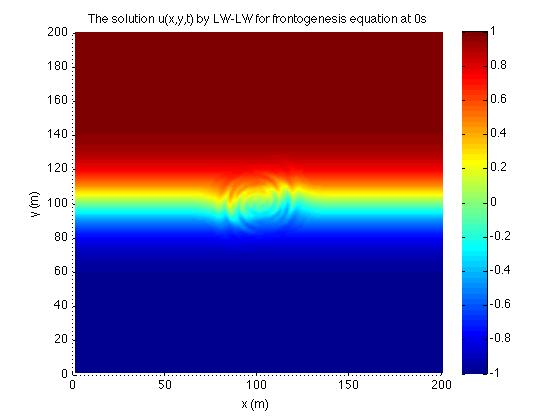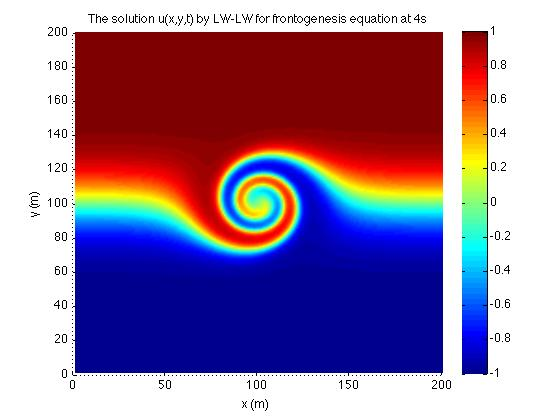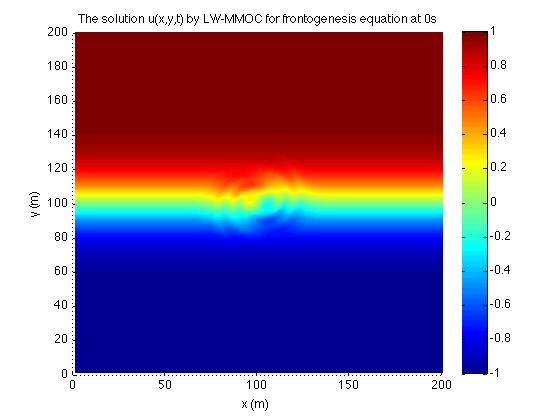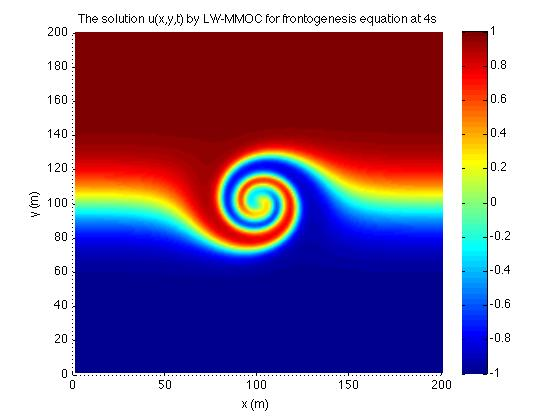Figure 1. The initial condition and target function by $LW$ (top) and MMOC (bottom).

Results of $CPU$ time and $L_2$ norm of the numerical initial condition with respect to the exact initial condition are displayed in Table . The results are quite the same for the $LW$ and $MMOC$, since we have a linear transport equation.

$Scheme$ $CPU~ time~ (s)$ $L_2~ norm$
$LW$ 49.0 4.07
$MMOC$ 80.8 3.96
Table 1. Performance for initial condition in the Doswell frontogenesis.

### Inviscid Burgers equation

We consider the Burgers equation with zero viscosity term which is called the inviscid Burgers equation. This equation is one of the most useful formulation of the behaviour of the shock waves in which nonlinear advection can be observed. In this nonlinear problem the flux function is

\begin{equation} f (u) = \frac{1}{2} u^2 . \end{equation}

The exact solution of this problem is given by

\begin{equation} u(x,t) = sin(x - ut) . \end{equation}

The domain $\Omega = [0,32]^2$ is discretized in 4096 square cells, a step size $\epsilon = 0.01$ is selected and the Courant number is set to $CFL = 0.1$. The exact and $MMOC$ initial conditions are shown in Fig 2 considering zero values as a guess initial condition. Only with the $MMOC$ scheme the numerical solver was able to converge to the initial condition for the selected step size.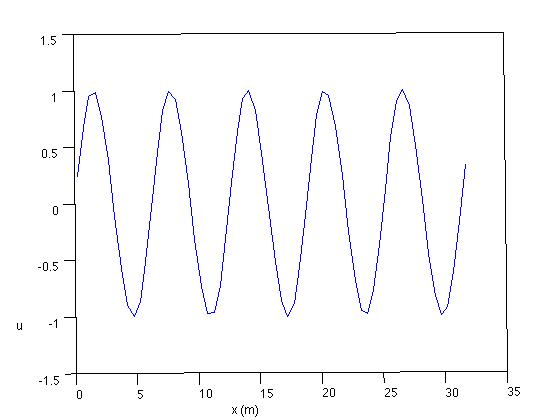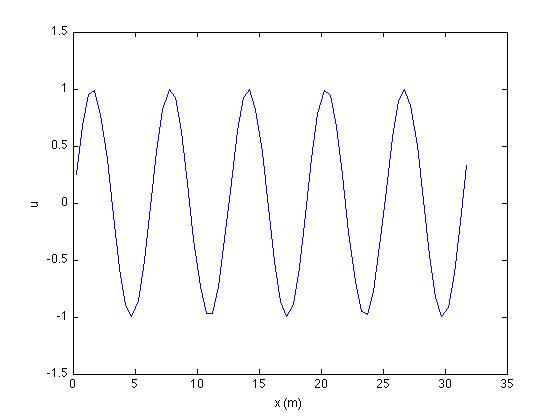Figure 2.The exact (left) and $MMOC$ (right) initial conditions.

In order to observe the convergence to the initial condition, we set $u_0(x) = \beta sin(x)$ as a guess initial condition, where $\beta$ is a constant. As $\beta$ is next to one, the guess initial conditions is closer to the exact initial condition. Table 2 displays the $CPU$ time and $L_2$ norm of the numerical initial condition with respect to the exact initial condition for several $\beta$ values. The numerical solver using $MMOC$ scheme converged for any $\beta$ value, showing that the norm is smaller as the $\beta$ is increased.

$\beta$ $LW$ $MMOC$
0.1 3.68 3.39
0.5 2.82 2.70
0.9 0.95 1.19
Table 2. Performance for initial condition by $MMOC$ in the Burgers problem.

# Conclusions

The results achieved and presented here are preliminary ones. The $MMOC$ is a promising eulerian-lagrangian scheme for the problem of inverse design of nonlinear transport equations. More complex problems and another eulerian-lagrangian scheme are to be tested. The next steps are the follows:

• simulate and investigate the Buckley-Leverett problem;
• simulate and investigate the miscible tracer flow; and
• use the $LCELM$ eulerian-lagrangian scheme .

 M. Morales-Hernandez, E. Zuazua, Adjoint computational methods for 2d inverse design of linear transport equations on unstructured grids, Computational and Applied Mathematics (2019)

 J. Douglas, T. F. Russell, Numerical methods for convection dominated diffusion problems based on combining the method of characteristics with finite element or finite difference procedures, SIAM J. Numer. Anal. (1982)

 J. Douglas, F. Pereira, L.-M. Yeh, A locally conservative euleriana lagrangian numerical method and its application to nonlinear transport in porous media, Computational Geosciences (2000)